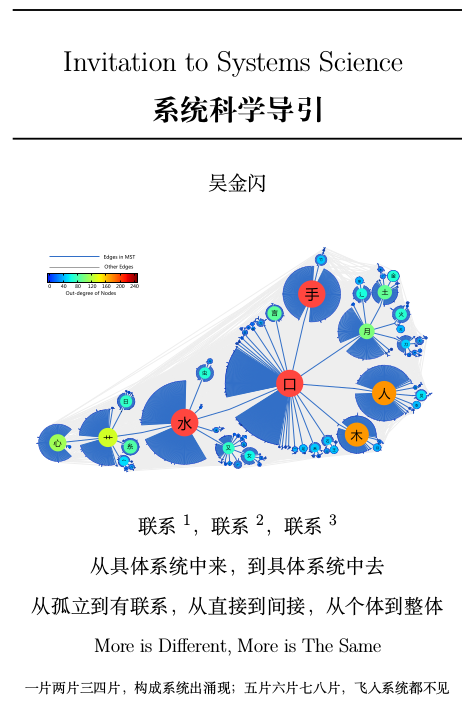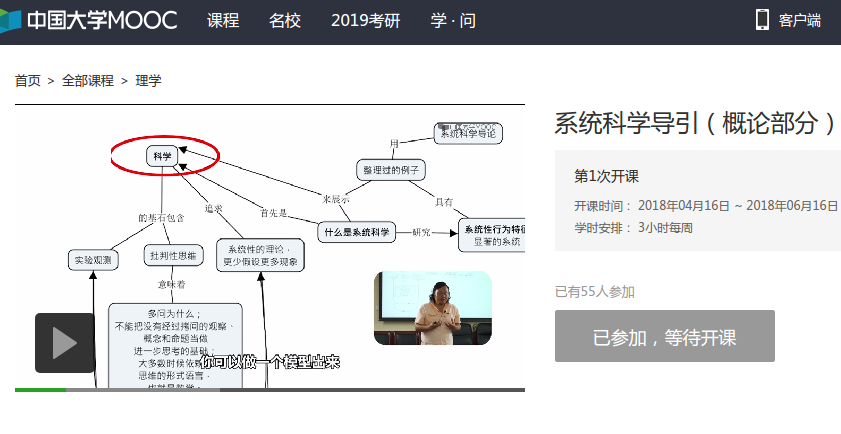## 《系统科学导引》序——方福康

《系统科学导引》序

（一）

(二)

(三)

2018年4月

 Norbert Wiener, I Am a Mathematician: The Later Life of a Prodigy, 1964, p.324.
 徐光宪，化学分子信息量的计算和可见宇宙信息量的估，中国科学 B 辑：化学，2007年，第 37 卷， 第 4 期：313-31.
 达尔文，《人类的由来》，第三章，1887.
 Martin A. Nowak，Evolutionary Dynamics，Harvard University Press，2006.

## 《系统科学导引》图书和MOOC课程上线了1. 从科学而不是哲学和数学的角度来讨论系统科学
• 批判性思维、系联性思考、可重复性V.S.可证伪性的讨论
• 科学（物理学、系统科学）和数学的关系
• 赏析大量的具体科学研究工作来体现什么是系统科学
2. 系统科学的思维方式和分析方法的提炼总结
• 系联：从孤立到有联系，从直接到间接，从个体到整体
• 分析与综合或者说还原论和整体论的结合
• 交叉性：从具体问题中来，到具体问题中去，留下可能的一般性理论## 第二届系统科学大会《系统科学导引》专题讨论邀请

————————————

Title: 系统科学基本教材建设讨论，以《系统科学导引》为例
Corresponding organizer: 吴金闪 (51362)
Organizers with PINs: 吴金闪 (51362)
Abstract:
《系统科学导引》已经在出版过程中。在这个专题讨论中中，我将介绍这本书的主要内容、设计思想，以及讨论一些例子，来跟大家展开对这本书的讨论。在此之前，我会把电子版和纸质版的书送到感兴趣的参会者手上。以这本书为例，这个小组讨论将展开对系统科学的基本教材建设的讨论。除了对本书进一步修改、推广的意见和建议之外，这个小组讨论还争取能够在本书基础上形成本学科的基本教材的撰写计划。

More is Different, More is The Same（一片两片三四片，构成系统出涌现；五片六片七八片，飞入系统都不见）

Keywords:
《系统科学导引》、学科建设、教材建设

————————————

## 概念网络上的高效学习方式

1. 给定$$\vec{S}$$之后在第$$m$$步的累计成本为
2. \begin{align}
C\left(\vec{S}, m\right) = \sum_{j=1}^{m} C_{s_{j}}
\end{align}

3. 给定$$\vec{S}$$之后在第$$m$$步的累计价值为
4. \begin{align}
V\left(\vec{S}, m\right) = \sum_{j=1}^{m} V_{s_{j}}
\end{align}

1. 认识下层字$$j$$，以一定的概率降低上层字$$i$$的学习成本，$$\omega^{j, \left(\uparrow\right)}_{i}$$，和结构矩阵的元素$$a^{j}_{i}$$有关。原则上可以不遵循结构矩阵，来自于其他实证关系。做为一个简化模型，我们可以假设$$\omega^{j, \left(\uparrow\right)}_{i} = 0 \mbox{或者} a^{j}_{i}$$。更复杂的需要考虑理据性。
2. 认识上层字$$j$$，以一定的概率降低下层字$$i$$的学习成本$$\omega^{j, \left(\downarrow\right)}_{i}$$，和结构矩阵的元素$$a^{i}_{j}$$有关。原则上可以不遵循结构矩阵，来自于其他实证关系。做为一个简化模型，我们可以假设$$\omega^{j, \left(\downarrow\right)}_{i} = 0$$。更复杂的需要考虑理据性。
3. 有了这个改变学习成本的概率，我们再来看学习成本改变的值。采用递归定义：
4. \begin{align}
c_{i}\left(t\right) = \left(1-k_{i}\right) \cdot \left[\Pi_{u}\left(1-k_{u}\omega^{u,\left(\downarrow\right)}_{i}\right)a^{i}_{u}\right]\cdot \left\{1+\sum_{d}\left(1-k_{d}\omega^{d\left(\uparrow\right)}_{i}\right) \cdot \left[1+\left(1-k_{d}\right)c_{d}\left(t\right)\right]a^{d}_{i}\right\}.
\end{align}

\begin{align}
c_{i}\left(t\right) = \left(1-k_{i}\right) \cdot \left\{1+\sum_{d}\left[1+\left(1-k_{d}\right)c_{d}\left(t\right)\right]a^{d}_{i}\right\}.
\end{align}